Impact Factor 3.498 | CiteScore 3.3
More on impact ›

# Frontiers in Earth Science## ORIGINAL RESEARCH article

Front. Earth Sci., 12 May 2021 | https://doi.org/10.3389/feart.2021.652344

# Experimental Study on Petrophysical Properties as a Tool to Identify Pore Fluids in Tight-Rock Reservoirs

• 1School of Earth Sciences and Engineering, Hohai University, Nanjing, China
• 2WA School of Mines: Minerals, Energy and Chemical Engineering, Curtin University, Perth, WA, Australia
• 3Department of Geophysics, Istituto Nazionale di Oceanografia e di Geofisica Sperimentale (OGS), Trieste, Italy
• 4Exploration and Development Research Institute of Changqing Oilfield, PetroChina, Xi'an, China

The petrophysical properties can be proper indicators to identify oil and gas reservoirs, since the pore fluids have significant effects on the wave response. We have performed ultrasonic measurements on two sets of tight siltstones and dolomites at partial saturation. P- and S-wave velocities are obtained by the pulse transmission technique, while attenuation is calculated using the centroid-frequency shift and spectral-ratio methods. The fluid sensitivities of different properties (i.e., P- and S-wave velocities, impedances and attenuation, Poisson's ratio, density, and their combinations) are quantitatively analyzed by considering the data distribution, based on the crossplot technique. The result shows that the properties (P- to S-wave velocity and attenuation ratios, Poisson's ratio, and first to second Lamé constant ratio) with high fluid-sensitivity indicators successfully distinguish gas from oil and water, unlike oil from water. Moreover, siltstones and dolomites can be identified on the basis of data distribution areas. Ultrasonic rock-physics templates of the P- to S-wave velocity ratio vs. the product of first Lamé constant with density obtained with a poroelastic model, considering the structural heterogeneity and patchy saturation, are used to predict the saturation and porosity, which are in good agreement with the experimental data at different porosity ranges.

## Introduction

The rock-physics properties are affected by the reservoir characteristics (i.e., lithology, porosity, permeability, and pattern) and the presence of pore fluids. Understanding the corresponding variations is essential for seismic exploration. Although these variations have been extensively investigated for intermediate- to high-porosity fluid-saturated rocks, the effects of partial saturation on low-porosity tight rocks are not well-understood and a fluid-sensitivity analysis can be useful to interpret the porosity-based saturation logs and related seismic data.

A set of fluid-sensitive identification factors (rock-physics properties and their combinations) have been proposed to identify pore fluids (e.g., Fatti et al., 1994; Smith and Sutherland, 1996; Goodway, 2001; Dillon et al., 2003; Qiao and An, 2007; Qi et al., 2017; Zeng et al., 2017). For example, Ostrander (1984) and Carcione and Cavallini (2002) showed that Poisson's ratio of gas-saturated rocks is lower than those of brine- and oil-saturated ones. Zhou and Hilterman (2010) used three seismic attributes to predict pore fluid and lithology and showed that their sensitivities are not significantly affected by the scale factors. Moreover, Pei et al. (2010) proposed a new fluid identification factor, which is more effective than the amplitude versus offset (AVO) attributes in low-porosity reservoirs. However, these methods are incomplete due to the lack of statistical analysis of data distribution, and most of the methods have been applied to detect gas reservoirs.

The problem is that a single physical property is insufficient to predict the reservoir features and fluid content, especially for the tight rocks. A set of properties should be used, because P and S waves respond differently to variations in fluid saturation. Previous studies show that P- and S-wave velocity and attenuation ratios (and ${Q}_{\mathrm{\text{P}}}^{-1}/{Q}_{\mathrm{\text{S}}}^{-1}$) are more sensitive to partial gas–water saturation (Murphy, 1982; Winkler and Nur, 1982; Klimentos, 1995; Chichinina et al., 2009; Qi et al., 2017). For example, Murphy (1982) showed that P-wave attenuation is more robust than S-wave attenuation in partially saturated Massillon sandstone, but the difference is small at full water saturation. Winkler and Nur (1982) found that the ratio of P- to S-wave attenuation is more sensitive to gas saturation than the P- to S-wave velocity ratio, showing that ${Q}_{\mathrm{\text{P}}}^{-1}/{Q}_{\mathrm{\text{S}}}^{-1}\le 1$ for water-saturated rocks and ${Q}_{\mathrm{\text{P}}}^{-1}/{Q}_{\mathrm{\text{S}}}^{-1}>1$ for gas-saturated rocks. These relations can be useful, but they have been applied to intermediate- and high-porosity rocks. Only a few studies deal with tight-rock reservoirs (e.g., Pei et al., 2010; Xue et al., 2013; Han et al., 2017; Qi et al., 2017; Pang et al., 2019). They mainly distinguish gas from liquid-saturated rocks for sandstone and shale reservoirs. However, the relations were hardly analyzed for tight siltstone and dolomite reservoirs.

The challenge is to propose a suitable theoretical model that can predict the elastic properties and wave response characteristics in partially saturated rocks. Seismic wave velocity dispersion and attenuation are considered to be caused by the wave-induced fluid flow mechanism (Mavko and Nur, 1979; Murphy, 1982; Winkler and Nur, 1982; Müller et al., 2010). Different poroelastic models have been developed to predict the velocity and attenuation observed in the laboratory and in the field data (Biot, 1956; White, 1975; Pride et al., 2004; Gurevich et al., 2010; Ba et al., 2017; Guo and Gurevich, 2020). Based on these models, rock-physics templates (RPTs) can be used to estimate porosity and saturation (Liu et al., 2015; Pang et al., 2019, 2020). Studies showed that RPTs, built with acoustic impedance and P- to S-wave velocity ratio, serve as tools for lithology and fluid identification (Chi and Han, 2009; Datta Gupta et al., 2012; Ba et al., 2013). RPTs based on seismic attenuation also describe the effect of partial saturation, porosity, and permeability (Dvorkin and Mavko, 2006; Picotti et al., 2018). The Gassmann's equation is mainly used to conduct fluid substitution in generating RPTs and obtain the elastic modulus of the saturated rock, but neglecting the effect of patchy saturation. The tight-rock reservoirs usually have complex structures and highly variable saturation due to the complex rock patterns, and we used a double double-porosity (DDP) model, considering the structural heterogeneity and the uneven patchy saturation, for fluid substitution in the RPTs.

The rock-physics properties and ultrasonic waveforms can be obtained at different fluid-saturated conditions from the laboratory measurements. In this study, we performed ultrasonic measurements on tight siltstones and dolomites, where the P- and S-wave velocities and attenuation are obtained at in situ confining pressure. These properties and their combinations are then evaluated by means of fluid-sensitive indicators (FSIs) and crossplots are shown to validate their effectiveness. Finally, RPTs are built by using the DDP model of Ba et al. (2017) to match the experimental data.

## Experiments

Two sets of tight rocks are collected from the reservoirs. One set of 12 siltstones are collected from the oil reservoirs of the Qingshankou Formation, Northeast China, whereas another set of 13 dolomites are collected from Ordovician and Cambrian Formations, West China. The porosities and permeabilities of siltstones range from 2.88 to 13.97% and 0.0045 to 0.39 mD and those of dolomites from 4.99 to 16.87% and 0.075 to 162.753 mD, respectively. The corresponding parameters are listed in Tables 1, 2, sorted from smallest to largest based on porosity. The low permeability in siltstones is due to the high clay content and fine texture, while the highly variable permeability in dolomites indicates the presence of fractures. More details on the rock properties can be found in the study by Ba et al. (2019).

TABLE 1
TABLE 2

The systematic ultrasonic measurements have been performed on the two sets of rocks at different saturations and in situ confining pressure. Siltstones were measured at a confining pressure of 50 MPa and a pore pressure of 25 MPa, whereas dolomites at 80 and 10 MPa, respectively. The adopted saturation method is described in the studies by Ba et al. (2017) and Ma and Ba (2020). The partially gas–water and oil–water saturation tests were performed on the dolomites, but we conducted these tests only in five siltstones, and we set full saturation in others (gas, water, and oil, respectively). The waveforms of P and S waves were recorded by the pulse transmission technique, and the corresponding velocities were calculated on the basis of the arrival time of the first high-amplitude signals. P- and S-wave attenuation were estimated by using one wave period with the centroid-frequency shift and spectral-ratio methods (Toksöz et al., 1979; Quan and Harris, 1997). The detailed measuring procedures and error analysis are given in the study by Ma and Ba (2020).

## Fluid-Sensitive Indicator and Petrophysical Properties

To quantitatively evaluate the sensitivity when the rocks are saturated with different fluids, an indicator (I) has been introduced by Pei et al. (2010) and Guo et al. (2015):

where $\overline{{X}_{i}}$ and $\overline{{X}_{\mathrm{\text{w}}}}$ are the average values of measured data at gas/oil and water saturations, respectively. A high I indicates that the property is more sensitive to the change of pore fluid. However, I values of different rocks can be similar and the data distribution pattern must be considered. A coefficient of dispersion (CD), which reflects the distribution range, is given by the following equation:

where SDi denotes the standard deviation for the gas/oil-saturated rocks and n is the number of samples. For rocks with partial saturation, $\overline{{X}_{i}}$ and SDi are the average and standard deviations, respectively. A small CD indicates that the data distribution is focused around the average value. Thus, the FSI is defined as follows:

The larger the FSI value, the higher the fluid detection capability.

Both the pore fluid and the characteristics of the rock frame affect the bulk modulus, while the fluid has almost no effect on the shear modulus. P- and S-wave velocities (VP, VS), attenuations (${Q}_{\mathrm{\text{P}}}^{-1}$, ${Q}_{\mathrm{\text{S}}}^{-1}$), and density (ρ) are obtained from the laboratory measurements. Other properties can be obtained from these properties described above, such as VP/VS, impedances Zp and Zs, Poisson's ratio ν, Young's modulus E, first and second Lamé constants λ, μ, λρ, λ/μ, and ${Q}_{\mathrm{\text{P}}}^{-1}/{Q}_{\mathrm{\text{S}}}^{-1}$. These 14 properties, except μ, are used for the fluid-sensitivity analysis.

## Results

The I, CD, and FSI values of the above-mentioned properties are reported in Table 3. Figure 1 shows the indicators for siltstones, where it can be seen that I corresponding to ${Q}_{\mathrm{\text{P}}}^{-1}/{Q}_{\mathrm{\text{S}}}^{-1}$ is the most sensitive and those of λ, λρ, λ/μ, and attenuation are high (Figure 1A). This is due to the fact that attenuation is more sensitive to the changes in fluid properties and saturation. The FSI values of VP/VS, ν, and λ/μ are the highest when their CD values are the lowest (Figures 1B,C), i.e., the most sensitive to fluid-type variations. Since P and S waves respond differently to the variations of rock and fluid properties, the S-wave properties can be used as normalization quantities with which we compared P-wave properties, to remove in part the effect of rock frame. Moreover, they can better distinguish gas from water than oil from water. Although the I values of ${Q}_{\mathrm{\text{P}}}^{-1}/{Q}_{\mathrm{\text{S}}}^{-1}$ are the highest, the data distribution is scattered due to the complex fluid distribution, resulting in low FSI values. Figure 2 shows the I, CD, and FSI values for the dolomites, which are similar to those of siltstones.

TABLE 3
FIGURE 1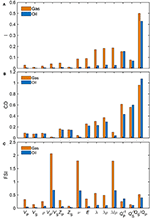Figure 1. (A) I, (B) CD, and (C) FSI for siltstones at full saturation. The brown and blue rectangles denote the values of fluid sensitivity to distinguish gas from water and oil from water, respectively.

FIGURE 2

The crossplot technique is used to verify the fluid identification capability of the properties with the high indicators I and FSI. Figures 3A,B shows the crossplots between λ vs. λρ in siltstones and dolomites, respectively, indicating that the fluid discrimination is difficult. Figures 3C,D, which displays crossplots of λρ vs. λ/μ, shows that the gas-saturated samples can be identified from those saturated with water and oil, but the water and oil cannot be distinguished. In fact, λ/μ has smaller values and significant variations with gas saturation, from 1 to 1.4 for siltstones and ~from 0.8 to 1.1 for dolomites. Figures 3E,F shows the similar trends of λ/μ and ${Q}_{\mathrm{\text{P}}}^{-1}/{Q}_{\mathrm{\text{S}}}^{-1}$as Figures 3C,D, but the data points are widely distributed and have poor convergence. Figure 4 shows the crossplots of ν, λ/μ, ${Q}_{\mathrm{\text{P}}}^{-1}/{Q}_{\mathrm{\text{S}}}^{-1}$, and VP/VS with high FSI values. There is a linear relation between ν, λ/μ, and VP/VS (Figures 4A–D), indicating that these properties have a similar variation trend when the pore fluid changes. Gas saturation can be identified from water saturation, while the data for full oil and water saturation are mixed. Figures 4E,F indicates that the crossplots of VP/VS and ${Q}_{\mathrm{\text{P}}}^{-1}/{Q}_{\mathrm{\text{S}}}^{-1}$ discriminate between gas and water or oil. The properties with high FSI values show a better fluid identification capability than those with high I values, showing that the data distribution characteristic is important when quantifying the fluid sensitivity.

FIGURE 3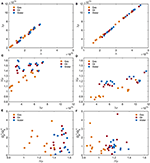Figure 3. Crossplots of λρ –λ, λ/μ –λρ, and ${Q}_{\mathrm{\text{P}}}^{-1}\mathrm{\text{/}}{Q}_{\mathrm{\text{S}}}^{-1}$–λ/μ for siltstones (A,C,E) and dolomites (B,D,F). The brown, red, and blue points denote the data at full gas, oil, and water saturation, respectively. The units of λ and λρ are kg/m3 × (m/s)2 and (kg/m3 × m/s)2, respectively.

FIGURE 4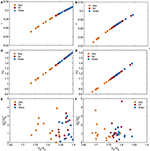Figure 4. Crossplots of ν –VP/VS, λ/μ –VP/VS, and ${Q}_{\mathrm{\text{P}}}^{-1}\mathrm{\text{/}}{Q}_{\mathrm{\text{S}}}^{-1}$VP/VS for siltstones (A,C,E) and dolomites (B,D,F) (see Figure 3 for the explanations).

Next, we studied the fluid sensitivity in partially saturated rocks (gas–water or oil–water). Figure 5 shows that VP/VS, ν, and λ/μ are high, in agreement with the full saturation case. Since these properties have a similar variation trend, we only obtained the crossplots of VP/VS and λ/μ, and are shown in Figures 6A,B. They decrease with increasing gas saturation and distinguish between partial and full saturation. However, the data cannot reflect the petrophysical property variation with the variations in reservoir property (i.e., porosity). The λρ and ${Q}_{\mathrm{\text{P}}}^{-1}/{Q}_{\mathrm{\text{S}}}^{-1}$ are used, which have been verified at full saturation. Figures 6C,D shows that VP/VS vs. λρ decreases with gas saturation, while ${Q}_{\mathrm{\text{P}}}^{-1}/{Q}_{\mathrm{\text{S}}}^{-1}$ increases (Figures 6E,F). Similar results have been obtained in the studies by Murphy (1982) and Winkler and Nur (1982). Figure 7 shows the crossplots for partially oil–water saturated rocks. These properties have smaller variations with oil saturation than in the case of gas, and it is difficult to distinguish oil from water. However, the data of siltstones and dolomites have different distribution areas, which can be well-discriminated using the crossplots.

FIGURE 5
FIGURE 6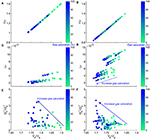Figure 6. Crossplots of λ/μ –VP/VS, λρ –VP/VS, and ${Q}_{\mathrm{\text{P}}}^{-1}\mathrm{\text{/}}{Q}_{\mathrm{\text{S}}}^{-1}$VP/VS for siltstones (A,C,E) and dolomites (B,D,F) at partial gas–water saturation. The color from green to blue denotes gas saturation increasing from 0 to 100%.

FIGURE 7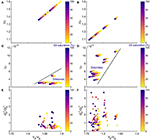Figure 7. Crossplots of λ/μ –VP/VS, λρ –VP/VS, and ${Q}_{\mathrm{\text{P}}}^{-1}\mathrm{\text{/}}{Q}_{\mathrm{\text{S}}}^{-1}$VP/VS for siltstones (A,C,E) and dolomites (B,D,F) at partial oil–water saturation. The color from yellow to purple denotes oil saturation increasing from 0 to 100%.

The RPTs are the useful tools in petroleum exploration, relating reservoir, fluid, and petrophysical properties. In this study, we used the DDP model developed by Ba et al. (2017) (see Appendix A), which considers the fabric structure heterogeneity and uneven patchy saturation to build the templates at ultrasonic frequencies. Siltstones mainly consist of feldspar, quartz, and clay, and we considered clay with intragranular pores as the inclusion with a mean radius of 40 μm embedded in the host medium. Porosity and saturation are set as independent variables. The grain bulk and shear moduli are set to 39 and 31 GPa, respectively, estimated by the average Voigt–Reuss–Hill equation (Hill, 1952; Picotti et al., 2018), while the grain density is 2.6 g/cm3. The dry-frame and inclusions moduli vs. porosity are calculated by the differential effective medium model (Mavko et al., 2009). The fluid properties at the experimental conditions are obtained by the equations from Batzle and Wang (1992), which are reported in the study by Ba et al. (2019), and the permeability is given by the Kozeny–Carman relation (e.g., Mavko et al., 2009). Figure 8 shows the template at 1 MHz, compared with the measured data of VP/VS and λρ. The black and red curves denote the isolines of constant saturation and porosity, respectively. The figure shows that the predicted porosities (red lines) agree with the sample porosities. For samples I–L with relatively high porosities (10.87–13.97%), the data agree well with the predicted saturation (black lines) at high gas saturations, but the predictions underestimate the data at low gas saturation. The data corresponding to sample H do not match the gas saturation, which may be due to the fact that frame properties of this sample (6.45% porosity) have a significant difference from those of samples I–L.

FIGURE 8

The dolomites contain cracks and intergranular pores. Soft cracks are inclusions and have a mean radius of 80 μm. The density, bulk modulus, and shear modulus of the mineral grains are 2.7 g/cm3, 78 and 52 GPa, respectively. Figure 9A shows the ultrasonic template compared with the experimental data, from which it can be noted that the porosities and saturation of samples DO2–6 (4.99–6.93%) agree with the template predictions. However, samples DO7–13 with a porosity ranging from 10.37 to 16.87% are outside the template even if the predicted porosities match the data. The data between samples DO1–6 and DO7–13 are dissimilar, and the reason may be the different frame properties at relatively high porosities compared with the low porosity case. Then, we recalculated the template by changing the bulk and shear moduli of the mineral grains to 55 and 50 GPa, respectively (Appendix B). The results show that the porosities and saturations of samples DO7–13 agree with the template in Figure 9B. Some data points are outliers owing to the complex pore structures and fluid distribution. Nevertheless, the present template describes the general trends of porosity and saturation.

FIGURE 9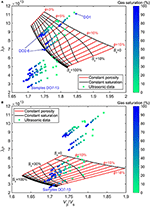Figure 9. Comparisons between the ultrasonic template and measured data for dolomites. (A) Template for samples DO1–6 with low porosities (4.99–6.93%). (B) Template for samples DO7–13 with relatively high porosities (10.37–16.87%).

Other models (e.g., White, 1975; Pride et al., 2004; Gurevich et al., 2010; Müller et al., 2010) can also be used to build RPTs, but most of them only consider the fabric (pattern) or fluid heterogeneity. Our model considers the effect of the two heterogeneities. Based on the templates of VP/VS and λρ combined with the experimental data, gas can be identified from oil and water at the ultrasonic frequency band. However, the templates of ${Q}_{\mathrm{\text{P}}}^{-1}/{Q}_{\mathrm{\text{S}}}^{-1}$ and VP/VS cannot be built because S-wave attenuation is not predicted by the model. Moreover, we have to consider an upscaling of attenuation to lower (seismic) frequencies. In practical applications, the seismic template can be obtained by combining the laboratory, sonic, and seismic data (e.g., Pang et al., 2019, 2020).

## Conclusion

We have studied the fluid sensitivity of petrophysical properties based on the experimental data by calculating fluid indicators and using crossplots. The FSI considering the data distribution in quantifying the sensitivity is better than the I indicator. The crossplots of ν, λ/μ, ${Q}_{\mathrm{\text{P}}}^{-1}/{Q}_{\mathrm{\text{S}}}^{-1}$, and VP/VS discriminate well between gas and liquids (i.e., water and oil). The siltstone and dolomite data can be identified based on the data distribution areas. As a prediction tool, a DDP theory has been used to build RPTs between VP/VS and λρ and to obtain gas saturation and porosity from the ultrasonic data. Moreover, the frame properties of dolomites may greatly vary at relatively high porosities compared with the low porosity case.

## Data Availability Statement

The original contributions presented in the study are included in the article/Supplementary Material. Further inquiries can be directed to the corresponding author.

## Author Contributions

JB conceived and designed the experiments. RM analyzed the experimental data. RM and JB wrote the manuscript. JB, JC, ML, and CW provided critical revisions of the manuscript. All authors contributed to the article and approved the submitted version.

## Funding

This work was supported by the Jiangsu Province Science Fund for Distinguished Young Scholars (BK20200021), the National Science and Technology Major Project of China (2017ZX05069-002), the research funds from SINOPEC Key Laboratory of Geophysics, the Jiangsu Innovation and Entrepreneurship Plan, the National Natural Science Foundation of China (41974123, 41704109), and the Fundamental Research Funds for the Central Universities, and the China Scholarship Council (201906710129).

## Conflict of Interest

CW was employed by the company Petrochina.

The remaining authors declare that the research was conducted in the absence of any commercial or financial relationships that could be construed as a potential conflict of interest.

## Supplementary Material

The Supplementary Material for this article can be found online at: https://www.frontiersin.org/articles/10.3389/feart.2021.652344/full#supplementary-material

## References

Ba, J., Cao, H., Carcione, J. M., Tang, G., Yan, X.-F., Sun, W., et al. (2013). Multiscale rock-physics templates for gas detection in carbonate reservoirs. J. Appl. Geophys. 93, 77–82. doi: 10.1016/j.jappgeo.2013.03.011

Ba, J., Ma, R., Carcione, J. M., and Picotti, S. (2019). Ultrasonic wave attenuation dependence on saturation in tight oil siltstones. J. Petrol Sci. Eng. 179, 1114–1122. doi: 10.1016/j.petrol.2019.04.099

Ba, J., Xu, W., Fu, L.-Y., Carcione, J. M., and Zhang, L. (2017). Rock anelasticity due to patchy saturation and fabric heterogeneity: a double double-porosity model of wave propagation. J. Geophys. Res. Solid Earth 122, 1949–1976. doi: 10.1002/2016JB013882

Batzle, M., and Wang, Z. (1992). Seismic properties of pore fluids. Geophysics 57, 1396–1408. doi: 10.1190/1.1443207

Biot, M. A. (1956). Theory of propagation of elastic waves in a fluid-saturated porous solid. II. Higher frequency range. J. Acoust. Soc. Am. 28, 179–191. doi: 10.1121/1.1908241

Carcione, J. M., and Cavallini, F. (2002). Poisson's ratio at high pore pressure. Geophys. Prospect. 50, 97–106. doi: 10.1046/j.1365-2478.2002.00299.x

Chi, X., and Han, D. (2009). Lithology and fluid differentiation using a rock physics template. Lead. Edge 28, 60–65. doi: 10.1190/1.3064147

Chichinina, T., Obolentseva, I., Gik, L., Bobrov, B., and Ronquillo-Jarillo, G. (2009). Attenuation anisotropy in the linear-slip model: interpretation of physical modeling data. Geophysics 74, WB165–WB176. doi: 10.1190/1.3173806

Datta Gupta, S., Chatterjee, R., and Farooqui, M. Y. (2012). Rock physics template (RPT) analysis of well logs and seismic data for lithology and fluid classification in Cambay Basin. Int. J. Earth Sci. 101, 1407–1426. doi: 10.1007/s00531-011-0736-1

Dillon, L., Schwedersky, G., Vásquez, G., Velloso, R., and Nunes, C. (2003). A multiscale DHI elastic attributes evaluation. Lead. Edge 22, 1024–1029. doi: 10.1190/1.1623644

Dvorkin, J. P., and Mavko, G. (2006). Modeling attenuation in reservoir and nonreservoir rock. Lead. Edge 25, 194–197. doi: 10.1190/1.2172312

Fatti, J. L., Smith, G. C., Vail, P. J., Strauss, P. J., and Levitt, P. R. (1994). Detection of gas in sandstone reservoirs using AVO analysis: a 3-D seismic case history using the Geostack technique. Geophysics 59, 1362–1376. doi: 10.1190/1.1443695

Goodway, B. (2001). AVO and lamé constants for rock parameterization and fluid detection. CSEG Rec. 6, 39–60.

Guo, J., and Gurevich, B. (2020). Frequency-dependent P wave anisotropy due to wave-induced fluid flow and elastic scattering in a fluid-saturated porous medium with aligned fractures. J. Geophys. Res. Solid Earth 125:e2020JB020320. doi: 10.1029/2020JB020320

Guo, Y., Ma, H., Ba, J., Yu, H., and Long, C. (2015). Impact of data distribution on fluid sensitivity analysis: a quantitative investigation. J. Appl. Geophys. 119, 1–15. doi: 10.1016/j.jappgeo.2015.05.003

Gurevich, B., Makarynska, D., de Paula, O. B., and Pervukhina, M. (2010). A simple model for squirt-flow dispersion and attenuation in fluid-saturated granular rocks. Geophysics 75, N109–N120. doi: 10.1190/1.3509782

Han, X., Wu, E., Guo, J., Xu, D., Sun, Z., Luo, X., et al. (2017). Fluid factor index for fluid saturation estimation. J. Nat. Gas Sci. Eng. 39, 72–81. doi: 10.1016/j.jngse.2017.01.026

Hill, R. (1952). The elastic behaviour of a crystalline aggregate. Proc. Phys. Soc. A 65, 349–354. doi: 10.1088/0370-1298/65/5/307

Klimentos, T. (1995). Attenuation of P- and S-waves as a method of distinguishing gas and condensate from oil and water. Geophysics 60, 447–458. doi: 10.1190/1.1443782

Liu, Q., Yin, X., and Li, C. (2015). Fluid discrimination based on rock physics templates. J. Geophys. Eng. 12, 830–838. doi: 10.1088/1742-2132/12/5/830

Ma, R., and Ba, J. (2020). Coda and intrinsic attenuations from ultrasonic measurements in tight siltstones. J. Geophys. Res. Solid Earth 125:e2019JB018825. doi: 10.1029/2019JB018825

Mavko, G., Mukerji, T., and Dvorkin, J. (2009). The Rock Physics Handbook: Tools for Seismic Analysis of Porous Media, 2nd Edn. Cambridge; New York, NY: Cambridge University Press.

Mavko, G. M., and Nur, A. (1979). Wave attenuation in partially saturated rocks. Geophysics 44, 161–178. doi: 10.1190/1.1440958

Müller, T. M., Gurevich, B., and Lebedev, M. (2010). Seismic wave attenuation and dispersion resulting from wave-induced flow in porous rocks — a review. Geophysics 75, 75A147–75A164. doi: 10.1190/1.3463417

Murphy, W. F. (1982). Effects of partial water saturation on attenuation in Massilon sandstone and Vycor porous glass. J. Acoust. Soc. Am. 71, 1458–1468. doi: 10.1121/1.387843

Ostrander, W. J. (1984). Plane-wave reflection coefficients for gas sands at nonnormal angles of incidence. Geophysics 49, 1637–1648. doi: 10.1190/1.1441571

Pang, M., Ba, J., Carcione, J. M., Picotti, S., Zhou, J., and Jiang, R. (2019). Estimation of porosity and fluid saturation in carbonates from rock-physics templates based on seismic Q. Geophys. 84, M25–M36. doi: 10.1190/geo2019-0031.1

Pang, M., Ba, J., Fu, L.-Y., Carcione, J. M., Markus, U. I., and Zhang, L. (2020). Estimation of microfracture porosity in deep carbonate reservoirs based on 3D rock-physics templates. Interpretation 8, SP43–SP52. doi: 10.1190/INT-2019-0258.1

Pei, F.-G., Zou, C.-C., He, T., Shi, G., Qiu, G.-G., and Ren, K.-Y. (2010). Fluid sensitivity study of elastic parameters in low-medium porosity and permeability reservoir rocks. Appl. Geophys. 7, 1–9. doi: 10.1007/s11770-010-0005-2

Picotti, S., Carcione, J. M., and Ba, J. (2018). Rock-physics templates based on seismic Q. Geophysics 84, MR13–MR23. doi: 10.1190/geo2018-0017.1

Pride, S. R., Berryman, J. G., and Harris, J. M. (2004). Seismic attenuation due to wave-induced flow. J. Geophys. Res. Solid Earth 109:B01201. doi: 10.1029/2003JB002639

Qi, Q., Müller, T. M., and Pervukhina, M. (2017). Sonic QP/QS ratio as diagnostic tool for shale gas saturation. Geophysics 82, MR97–MR103. doi: 10.1190/geo2016-0499.1

Qiao, Y., and An, H. (2007). Study of petrophysical parameter sensitivity from well log data. Appl. Geophys. 4, 282–287. doi: 10.1007/s11770-007-0038-3

Quan, Y., and Harris, J. M. (1997). Seismic attenuation tomography using the frequency shift method. Geophysics 62, 895–905. doi: 10.1190/1.1444197

Smith, G. C., and Sutherland, R. A. (1996). The fluid factor as an AVO indicator. Geophysics 61, 1425–1428. doi: 10.1190/1.1444067

Toksöz, M. N., Johnston, D. H., and Timur, A. (1979). Attenuation of seismic waves in dry and saturated rocks: I. Laboratory measurements. Geophysics 44, 681–690. doi: 10.1190/1.1440969

White, J. E. (1975). Computed seismic speeds and attenuation in rocks with partial gas saturation. Geophysics 40, 224–232. doi: 10.1190/1.1440520

Winkler, K. W., and Nur, A. (1982). Seismic attenuation: effects of pore fluids and frictional-sliding. Geophysics 47, 1–15. doi: 10.1190/1.1441276

Xue, Y., Cao, J., Wang, D., Tian, R., and Shu, Y. (2013). Detection of gas and water using HHT by analyzing P- and S-wave attenuation in tight sandstone gas reservoirs. J. Appl. Geophys. 98, 134–143. doi: 10.1016/j.jappgeo.2013.08.023

Zeng, Q., Guo, Y., Jiang, R., Ba, J., Ma, H., and Liu, J. (2017). Fluid sensitivity of rock physics parameters in reservoirs: quantitative analysis. J. Seism. Explor. 26, 125–140.

Zhou, Z., and Hilterman, F. J. (2010). A comparison between methods that discriminate fluid content in unconsolidated sandstone reservoirs. Geophysics 75, B47–B58. doi: 10.1190/1.3253153

Keywords: tight rocks, experimental observation, petrophysical properties, fluid sensitivity, rock physics template, attenuation

Citation: Ma R, Ba J, Carcione J, Lebedev M and Wang C (2021) Experimental Study on Petrophysical Properties as a Tool to Identify Pore Fluids in Tight-Rock Reservoirs. Front. Earth Sci. 9:652344. doi: 10.3389/feart.2021.652344

Received: 12 January 2021; Accepted: 19 April 2021;
Published: 12 May 2021.

Edited by:

Pinbo Ding, China University of Petroleum, China

Reviewed by:

Lingyun Kong, University of North Dakota, United States
Junxin Guo, Southern University of Science and Technology, China
Zhiqi Guo, Jilin University, China

Copyright © 2021 Ma, Ba, Carcione, Lebedev and Wang. This is an open-access article distributed under the terms of the Creative Commons Attribution License (CC BY). The use, distribution or reproduction in other forums is permitted, provided the original author(s) and the copyright owner(s) are credited and that the original publication in this journal is cited, in accordance with accepted academic practice. No use, distribution or reproduction is permitted which does not comply with these terms.

*Correspondence: Jing Ba, jba@hhu.edu.cn; jingba@188.com